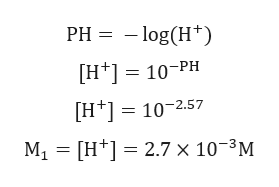# A certain benzoic acid solution has pH = 2.57. Calculate the volume of 0.0972 M KOH required to "neutralize" 15.8 mL of this solution.

Question
26 views

A certain benzoic acid solution has pH = 2.57. Calculate the volume of 0.0972 M KOH required to "neutralize" 15.8 mL of this solution.

check_circle

Step 1

Given:

PH of benzoic acid solution = 2.57

V1 = 15.8 mL.

Concentration of KOH = 0.0972 M

Step 2

Calculation for concentration o...help_outlineImage TranscriptionclosePH = – log(H*) [H*] = 10¬PH [H*] = 10-2.57 M1 = [H*] = 2.7 × 10-³M fullscreen

### Want to see the full answer?

See Solution

#### Want to see this answer and more?

Solutions are written by subject experts who are available 24/7. Questions are typically answered within 1 hour.*

See Solution
*Response times may vary by subject and question.
Tagged in

### Chemistry